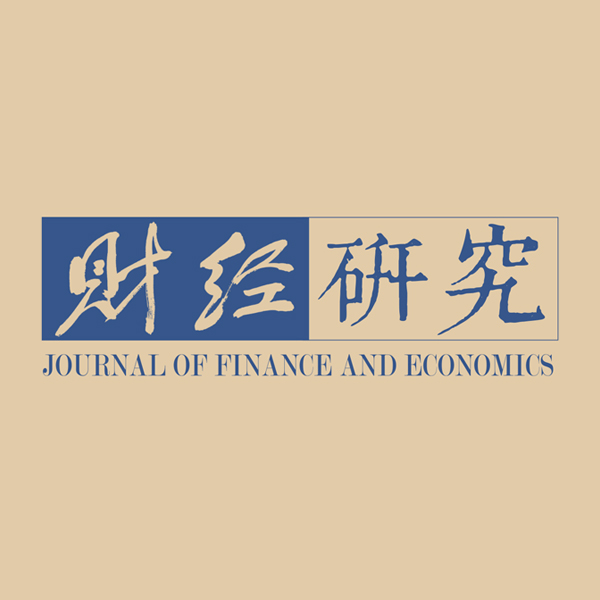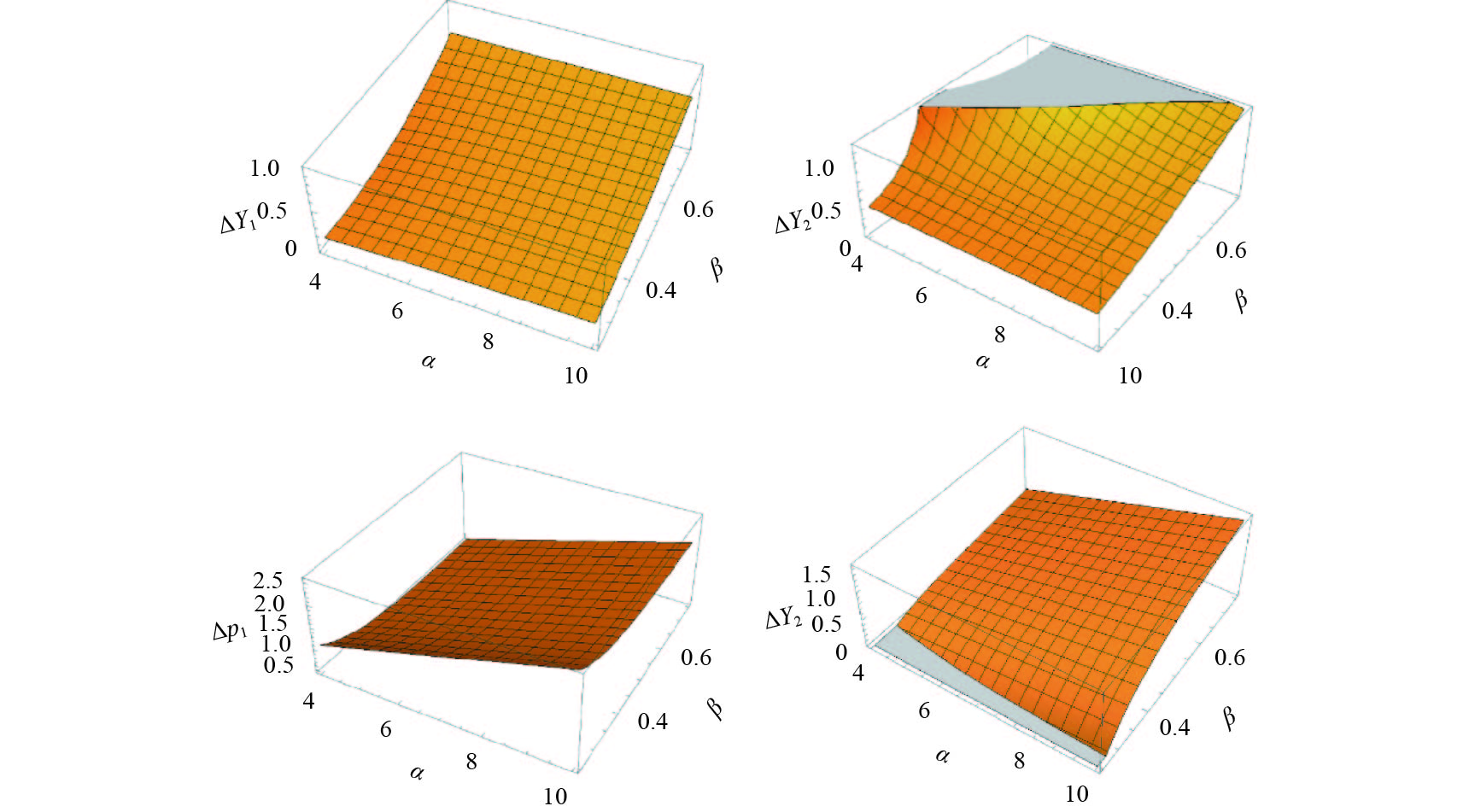﻿ “免费”商业模式下电商平台排他性行为研究《财经研究》
2019第45卷第6期
“免费”商业模式下电商平台排他性行为研究

1. 上海财经大学 商学院，上海 200433;
2. 上海财经大学 经济学院，上海 200433

A Research on the Exclusive Behavior of E-commerce Platforms under the “Free” Business Model
Zhang Qian1, Chen Qingzhu1, Chen Yifei21. College of Business，Shanghai University of Finance and Economics，Shanghai 200433，China;
2. School of Economics，Shanghai University of Finance and Economics，Shanghai 200433，China
Key words: exclusive behavior    business model    E-commerce platforms    two-sided markets

$k/4 \leqslant \beta \leqslant 3k/4$

$E{v_i}{\rm{ = }}\beta {d_i} + \left( {1 - {\gamma _i}} \right){p_i} - k\mathop \iint\limits_{\left( {x，y} \right) \in {\sigma _i}} {xydxdy} \geqslant 0$
。其中，σi表示接入平台i的卖方所属的区域（如图1所示）。（a）对应所有厂商均不实施排他性行为的情形，即σi={（xy）|0≤x≤1，0≤y≤1}。此时，卖方的预期成本为ECi=k/2。因此，在两个平台成本足够低的卖方会选择多归属，由（a）右下角灰色区域表示；在某一侧成本较低的卖方则会选择单归属，由图（a）左上角和右下角阴影区域表示；而在两个平台成本均较高的卖方则会选择不接入任何平台，由（a）中空白区域表示。相应地，（b）对应的是平台1实施排他性行为的情形，即σ1={（xy）|0≤x≤1，1/2≤y≤1}，σ2={（xy）|1/2≤x≤1，1/2≤y≤1}，得到其预期成本分别为EC1=k/2，EC2=k/4。此时，平台获得所有多归属用户（由灰色区域表示）。（c）对应了两个平台均实施排他性行为时的市场竞争结果，两个平台会平分市场，得到σi={（xy）|xy≤1，0≤x≤1}。相应地，卖方预期成本为ECi=3k/8。此时，原来的多归属用户群体中接入平台1较低的一半会选择平台1，而另一半则会选择平台2。图 1 卖方的接入成本及其归属行为

${u_i} = v + \alpha {s_i} - t{x_i} - {p_i}$
。由x1+x2=1，得到平台i上买方的数量：

 ${d_i} \equiv {x_i} = {1}/{2} +[{{{p_j} - {p_i} + \alpha \left( {{s_i} - {s_j}} \right)}}]/{{2t}},\;\;\left( {i,j \in \left\{ {1,2} \right\},i \ne j} \right)$ (1)

${v_i} = \beta {d_i} + \left( {1 - {\gamma _i}} \right){p_i} - k{C_i}$
。边际卖方确定价格以使得其期望效用为0，即Evi=0，又由ECi=1/2，得到：

 ${s_i} = \left[{\beta {d_i} + \left( {1 - {\gamma _i}} \right){p_i}}\right]/{k}$ (2)

 ${p_i} = \frac{{\left( {\beta - k} \right)\left[ {\beta - t\left( {1 - {\gamma _j}} \right)} \right]}}{{{\gamma _i}\left[ {\beta - t\left( {1 - {\gamma _j}} \right)} \right] + {\gamma _j}\left( {\beta - 2t} \right) + 2\left( {t - \beta } \right)}}$ (3)

${\pi _i} = {\gamma _i}{p_i}{d_i}$
。利用利润最大化的一阶条件，得到均衡中的价格抽成：

 $\gamma _i^* ={{[2\left( {t - \beta } \right)]/}}{{[k + 2\left( {t - \beta } \right)]}}$ (4)

 $p_i^* = {{[\left( {k - \beta } \right)\left( {k + 2t - 2\beta } \right)]}}/{{2k}}$ (5)

$\partial p_i^*/\partial \beta = - \left( {3k + 2t - 4\beta } \right)/2 < 0$
，表明卖方侧交叉网络外部性的提高会促使买方的支付价格下降。对于卖方而言，产品价格的变化会产生两种对冲效应：一方面，价格提高将通过增加卖方收入的方式提高其效用水平；另一方面，产品价格的提高将使得平台对买方缺乏吸引力，从而导致买方数量减少，并通过交叉网络外部性而促使卖方效用水平下降。由此，卖方侧交叉网络外部性的提高使得后者的影响超过了前者，从而卖方有动机降低产品要价。将公式（1）和（5）依次带入利润函数，得到：

 $\pi _i^* = {{[\left( {k - \beta } \right)\left( {t - \beta } \right)]}}/{{2k}}$ (6)

$\partial \pi _i^*/\partial k = \beta \left( {t - \beta } \right)/\left( {2{k^2}} \right) > 0$
，即成本参数的增加会提高平台的利润水平。

i.随着平台水平差异化程度的提高，均衡中的产品价格、价格抽成以及平台的利润水平均提高；

ii.随着卖方侧交叉网络外部性的增强，均衡中的产品价格、价格抽成及平台利润水平均降低；

iii.随着成本参数的提高，均衡中的产品价格提高，价格抽成下降，平台的利润水平提高；

iv.买方侧的交叉网络外部性不影响均衡结果。

1. 均衡分析。先考虑平台1实施排他性行为的情形。与基准模型不同，平台1的排他性行为使得成本足够低的卖方无法接入平台2（如图1所示）。从而卖方的期望效用函数变为：

 $E{v_1} = \beta {d_1} + \left( {1 - {\gamma _1}} \right){p_1} - k/2,\;E{v_2} = \beta {d_2} + \left( {1 - {\gamma _2}} \right){p_2} - k/4$ (7)

 ${p_1} = \frac{{4{\beta ^2} - \beta \left( {4t + \alpha + 3k - \alpha {\gamma _2} - 4{\gamma _2}t} \right) + 4kt\left( {1 - {\gamma _2}} \right)}}{{8t\left( {1 + {\gamma _1}{\gamma _2} - {\gamma _1} - {\gamma _2}} \right) - 4\beta \left( {2 - {\gamma _1} - {\gamma _2}} \right)}},\;{p_2} = \frac{{4{\beta ^2} - \beta \left( {4t + 3k + \alpha {\gamma _1} - 4{\gamma _1}t - \alpha } \right) + 2kt\left( {1 - {\gamma _1}} \right)}}{{8t\left( {1 + {\gamma _1}{\gamma _2} - {\gamma _1} - {\gamma _2}} \right) - 4\beta \left( {2 - {\gamma _1} - {\gamma _2}} \right)}}$ (8)

 ${\gamma _1}^\prime = 1 - \frac{{\beta \left( {3k - 4\beta } \right)}}{{\beta \left( {\alpha - 4t} \right) + 2tk}},{\gamma _2}^\prime = 1 - \frac{{\beta \left( {k - 4\beta } \right)}}{{8{\beta ^2} - \left( {12t + 4k - \alpha } \right)\beta + 4tk}}$ (9)

$\partial {\gamma _1}^\prime /\partial \alpha > 0,\partial {\gamma _2}^\prime /\partial \alpha < 0$
，即买方侧交叉网络外部性对两个平台价格抽成的影响是相反的。卖方侧交叉网络外部性对价格抽成的影响受到了{αβkt}的共同影响，因而是不确定的。将公式（9）关于平台差异化水平求偏导，并观察其符号变化情况可得
$sign(\partial {\gamma _1}^\prime /\partial t) = sign\left( {k - 2\beta } \right)$
$sign\left( {\partial {\gamma _2}^\prime /\partial t} \right) =$
$sign\left( {3\beta - k} \right)$
。由
$\beta \in \left[ {k/4,3k/4} \right]$

$\beta \in \left[ {k/3,k/2} \right]$

 ${p'_1} = {{[\alpha \beta + 2t\left( {k - 2\beta } \right)]}}/{{4\beta }},{p'_2} = 0$ (10)

$\partial {p'_1}/\partial \alpha = 1/4 > 0$
$\partial {p'_2}/\partial \beta = - kt/2{\beta ^2} < 0$
。买方侧交叉网络外部性的增强提高了买方对平台的依赖程度，从而促使产品价格提升；而卖方交叉网络外部性的增强则提高了平台1排他性行为的机会成本而使平台1的价格下降。将公式（1）、（9）和（10）代入利润函数得到：

 ${\pi '_1} = {{\left( {4\beta - k} \right)\left[ {4{\beta ^2} - \left( {4t + 3k - \alpha } \right)\beta + 2tk} \right]}}/{{16{\beta ^2}}},{\pi '_2} = 0$ (11)

$\partial {\pi '_1}/\partial \alpha = \left( {1 - k/4\beta } \right)/4 > 0$
$\partial {\pi '_1}/\partial \beta {\rm{ = 1 + }}k\left( {\alpha \beta - 3\beta k + 4kt - 12\beta t} \right)/16{\beta ^3}$
，即买方侧交叉网络外部性的增强会通过提高产品价格和价格抽成使平台1利润增加，卖方侧交叉网络外部性对平台1的利润影响是不明确的。当买方侧的交叉网络外部性足够强时，卖方侧交叉网络外部性的提高也会带来平台1利润的增加。另外，由利润最大化的二阶条件可得：

 $4{\beta ^2} - \left( {4t + 3k - \alpha } \right)\beta + 2tk > 0$ (12)

$\alpha > 2t\left( {k - 2\beta } \right)/\beta$
，由
$\beta \in \left[ {k/4,3k/4} \right]$

 $\alpha > 4t$ (13)

i.随着买方侧交叉网络外部性的增强，平台1的价格抽成提高，平台2的价格抽成降低；而卖方侧交叉网络外部性对平台价格抽成的影响是不确定的；

ii.随着买方（卖方）侧交叉网络外部性的增强，平台1上卖方的价格提高（下降）；而平台2上卖方的定价为0；

iii.平台1获得严格为正的利润，而平台2的利润为0。

2. 比较静态分析。为了分析平台单方面实施排他性行为的动机，现对比单边排他与基准模型两种均衡结果的相对变化。由于两种均衡的相对变化除受到参数{βkt}的影响外，还受到买方侧交叉网络外部性的影响。为了较为直观比较均衡结果的相对变化，令k=1。根据不等式条件

$k/4 < \beta < 3k/4$
$t \geqslant 3k/4$
$\alpha \geqslant 4t$
$4{\beta ^2} - \left( {4t + 3k - \alpha } \right)\beta + 2tk > 0$
，并通过对参数组合t赋值，从相对意义上考察卖方侧交叉网络外部性变化的比较静态结果。令Δx′≡x′−x*表示单边排他的均衡结果与基准模型的相对变化。不妨令t=1，得到不等式条件
$1/4 < \beta < 3/4,\alpha \geqslant 4$
，通过计算得到价格抽成、产品价格以及利润的相对变化情况（如图2所示）。图 2 单边排他与基准模型均衡结果对比

$\Delta {d'_1} = - \Delta {d'_2} = \left( {2\beta - 1} \right)/\left( {4\beta } \right)$
$\Delta {s'_1} = 0$
$\Delta {s'_2} = - 1/8 < 0$
$\Delta {p'_2} < 0$
$\Delta {\pi '_2} < 0$
。说明平台1实施排他性行为对买方数量的相对变化是不确定的。当卖方侧的交叉网络外部性较弱，即β<1/2时，平台1在卖方侧的数量优势无法充分转移到买方侧，从而平台2的价格优势吸引了更多买方接入，尽管此时平台2的利润为0；当卖方侧的交叉网络外部性较高，即β≥1/2时，平台1在卖方侧的数量优势通过交叉网络外部性转移到了买方侧，从而尽管平台1的要价较高，买方依旧会接入平台1。这使得平台1的利润水平高于基准模型对应的利润水平。据此，得到命题3。

i.

$\Delta {\gamma '_i} > 0$
，即平台的价格抽成相对于基准模型对应的价格抽成提高；

ii.

$\Delta {p'_1} > 0$
，即产品价格相对于基准模型中对应的价格水平提高；

iii.当

$\beta \geqslant \tilde \beta$

$\Delta {\pi '_1} \geqslant 0,\beta < \tilde \beta$
$\Delta {\pi '_1} < 0$
，即当卖方侧的交叉网络外部性强度较高时，平台1的利润高于基准模型；而当卖方侧的交叉网络外部性强度较低时，平台1的利润低于基准模型对应的利润水平。其中，
$\tilde \beta \in \left\{ {\beta |\Delta \pi '\left( {\beta {\rm{|}}\alpha \geqslant 4} \right) = 0} \right\}$

1. 均衡分析。在本文的框架下，当两个平台都提供排他性协议时，两个平台是对称的，两个平台拥有的卖方数量是相等的。根据图1，卖方的期望效用函数变为：

$E{v_i} = \beta {d_i} + \left( {1 - {\gamma _i}} \right){p_i} -$
$3k/8$
。同理，由公式（1）和（2）得到产品价格的表达式：

 ${p_i} = \frac{{\left( {4\beta - 3k} \right)\left( {\beta + {\gamma _2}t - t} \right)}}{{4\left[ {2t\left( {1 + {\gamma _1}{\gamma _2} - {\gamma _1} - {\gamma _2}} \right) - \beta \left( {2 - {\gamma _1} - {\gamma _2}} \right)} \right]}}$ (14)

 ${\gamma ''_i} = {{8\left( {t - \beta } \right)}}/{{[3k + 8\left( {t - \beta } \right)]}}$ (15)

 ${p''_i} = {{\left( {3k - 4\beta } \right)\left( {3k + 8t - 8\beta } \right)}}/{{24k}}$ (16)

$\partial {p''_i}/\partial k = \left( {3k - 4\beta } \right)/3k > 0$

 ${\pi ''_i} = \left( {3k - 4\beta } \right)\left( {t - \beta } \right) /{{6k}}$ (17)

i.平台的价格抽成随着卖方侧交叉网络外部性或成本参数的提高而下降，随着平台水平差异化程度的增强而提高；

ii.卖方的价格水平随着卖方侧交叉网络外部性的提高而下降，随着平台水平差异化程度或成本参数的增加而提高；

iii.平台的利润水平随着卖方侧交叉网络外部性的提高而下降，随着平台水平差异化程度或成本参数的增加而提高。

2. 比较静态分析。首先，令

$\Delta x'' \equiv x'' - {x^*}$

$k/4 < \beta < 3k/4$
$t \geqslant 3k/4$
，可知
$\beta < t$
。对比产品价格、平台的价格抽成以及平台利润的相对变化，得到：

 $\begin{array}{l} \Delta {{p''}_i} = {{\beta \left( {\beta - t} \right)}}/{{3k}} - {k}/{8} < 0,\Delta {{\gamma ''}_i} = {{2k\left( {t - \beta } \right)}}/[{{\left( {3k + 8t - 8\beta } \right)\left( {k + 2t - 2\beta } \right)}}] > 0,\\ \Delta {{d''}_i} = 0,\Delta {{s''}_i} = - 1/8,\Delta {{\pi ''}_i} = {{\beta \left( {\beta - t} \right)}}/{6} < 0 \end{array}$ (18)

i.产品价格低于基准模型中对应的价格水平；

ii.价格抽成高于基准模型中对应的水平；

iii.平台的利润低于基准模型中平台的利润水平。

1. 平台的排他性策略选择。根据对称性，只有平台2实施排他性行为时获得的利润与只有平台1实施排他时对应的利润相等，从而可以将平台i实施排他性行为时的均衡利润表示为πsi=π1。由命题3可得：在满足1/4<β<3/4，α≥4的约束条件下，当

$\beta \geqslant \tilde \beta$

$\beta < \tilde \beta$

${\beta \geqslant \tilde \beta }$
 平台1平台2 不排他 排他 不排他 ${\left( {\pi _1^{\rm{*}},\pi _2^{\rm{*}}} \right)}$ ${\left( {{\rm{0,\pi }}_2^{\rm{s}}} \right)}$ 排他 ${\left( {\pi _1^s,0} \right)}$ ${\left( {{{\pi ''}_1},{{\pi ''}_2}} \right)}$

$\beta \geqslant \tilde \beta$
）时，两个平台都会实施排他性行为，并使得竞争双方陷入“囚徒困境”；而当
$\beta < \tilde \beta$

2. 福利分析。接下来，本文对比不同均衡结果下的消费者剩余和社会福利的相对变化。其中，消费者剩余为买卖双方消费者剩余的总和，由买卖双方的效用函数可得：

 $CS = C{S_1} + C{S_2} = \mathop \sum \nolimits_1^2 \left[ {\mathop \int \nolimits_0^{{d_i}} {u_i}\left( x \right)dx + \mathop \int\!\!\!\int \nolimits_{\left( {x,y} \right) \in {\sigma _i}} {v_i}\left( {x,y} \right)dxdy} \right]$ (19)

i.

$C{S}''<C{S}'<C{{S}^{*}}$
，即消费者剩余在基准模型、单边排他、双边排他中依次下降；

ii.当

$1/2\mathop < \limits_\sim \beta \leqslant 3/4$

$W'' < W' < {W^*}$
，这说明当卖方侧的交叉网络外部性较强时，社会福利随着平台排他性的增加而降低；而当
$1/4 < \beta \mathop < 1/2$

$W'< W'' < {W^*}$

* 本文受上海财经大学研究生创新基金项目：平台竞争、规制与不对称信息（CXJJ-2016-334）；企业的搭售行为及其排他性效应研究（CXJJ-2016-356）资助。

① 来源：Hi商学院，天猫战略伙伴是什么（优势及要求），https://www.hishop.com.cn/ecschool/tm/show_20894.html，2015年5月20日。

② 来源：南方企业新闻网，天猫：战略合作伙伴超160个，20多个品牌独家合作，http://www.senn.com.cn/sc/2015/08/06-/67690.html，2015年08月06日。

③ “免费”商业模式是平台吸引用户、保持客户粘性的重要手段，并广泛存在于电商、即时通信、在线视频和搜索引擎等行业中。很多学者对“免费”商业模式进行了研究（Pauwels和Weiss，2008；袁宏伟，2010等），但是对“免费”商业模式的定义尚无统一界定。本文中，“免费”商业模式特指平台不对买方收费，而仅从卖方处获取价格抽成的模式。

④ 媒体型是指两侧用户不存在交易的双边市场类型，如报纸、广告等；而支付卡型则是指两侧用户之间存在交易的类型，如支付卡等。二者在理论上的差别依次体现为Armstrong（2006）提出的会员模型和Rochet和Tirole（2003及Rochet和Tirole2006）提出的使用模型。考虑电商平台的商业模式，本文对媒体型双边平台进行分析，而有关支付卡型双边平台的研究将在另一篇论文中进行研究。

⑤ 本文不对买方多归属的情形进行分析。主要是基于以下两个方面的考虑：一是在单位需求的假定下，买方不会同时在两个平台上购买产品，不具有多归属的动机。二是通过对买方多归属的情形进行计算，比较本文得到的结果与买方单归属的情形的均衡结果相差不大。

⑥ 该假定是根据基准模型中平台利润最大化的二阶条件得到的。同时，该条件能够保证价格水平和平台价格抽成非负。

⑦ 当买方（卖方）进行购买决策时，因效用水平受到卖方（买方）数量的影响，其自身的选择会对这一数量产生作用，从而在具有外部性的市场中广泛存在“鸡−蛋相生”问题。为了解决这一问题，多数研究假定用户对平台两侧用户数量的预期值与实现值相一致，这就是所谓的“可实现预期”（Katz和Shapiro，1985；Caillaud和Jullien，2003）。

⑧ 为了便于分析，本文平台的排他性行为不涉及平台为吸引卖方加入而提供的补贴。实际上，当考虑补贴时，实施排他性行为的平台需要对单归属卖方和多归属卖方进行区分以明确补贴对象。而为了最大化利润，平台将会在卖方接受排他性协议的前提下确定尽可能低的补贴水平，并防止单归属卖方为了获取补贴而选择多归属，从而平台的补贴水平将会降为0。

⑨ 本文还对t的其他赋值进行了计算，结果表明在上述约束条件下，t的高低仅影响结果的相对大小，而基本不影响相对结果的变化方向。

⑩ 由于

${k/4 < \beta < 3k/4}$

${ \ge 3k/4}$
，满足利润最大化的二阶条件：
${ \left( {3k - 4\beta } \right)\left( {t - \beta } \right) > 0}$

  高洁, 蒋传海, 王宇. 平台竞争与独家交易[J]. 财经研究, 2014(2): 67–74.  曲振涛, 周正, 周方召. 网络外部性下的电子商务平台竞争与规制——基于双边市场理论的研究[J]. 中国工业经济, 2010(4): 120–129.  袁宏伟. 基于互联网的" 免费”商业模式创新研究[J]. 商业研究, 2010(12): 192–196. DOI:10.3969/j.issn.1001-148X.2010.12.039  Aghion P, Bolton P. Contracts as a barrier to entry[J]. The American Economic Review, 1987, 77(3): 388–401.  Armstrong M. Competition in two-sided markets[J]. The RAND Journal of Economics, 2006, 37(3): 668–691. DOI:10.1111/(ISSN)1756-2171  Armstrong M, Wright J. Two-sided markets, competitive bottlenecks and exclusive contracts[J]. Economic Theory, 2007, 32(2): 353–380. DOI:10.1007/s00199-006-0114-6  Bernheim B D, Whinston M D. Exclusive dealing[J]. Journal of Political Economy, 1998, 106(1): 64–103. DOI:10.1086/250003  Caillaud B, Jullien B. Chicken & Egg: Competition among intermediation service providers[J]. The RAND Journal of Economics, 2003, 34(2): 309–328. DOI:10.2307/1593720  Chowdhury S M, Martin S. Exclusivity and exclusion on platform markets[J]. Journal of Economics, 2017, 120(2): 95–118. DOI:10.1007/s00712-016-0499-z  Hagiu A, Lee R S. Exclusivity and control[J]. Journal of Economics & Management Strategy, 2011, 20(3): 679–708.  Hałaburda H, Yehezkel Y. Platform competition under asymmetric information[J]. American Economic Journal: Microeconomics, 2013, 5(3): 22–68. DOI:10.1257/mic.5.3.22  Katz M L, Shapiro C. Network externalities, competition, and compatibility[J]. The American Economic Review, 1985, 75(3): 424–440.  Pauwels K, Weiss A. Moving from free to fee: How online firms market to change their business model successfully[J]. Journal of Marketing, 2008, 72(3): 14–31. DOI:10.1509/JMKG.72.3.014  Rasmusen E B, Ramseyer J M, Wiley Jr J S. Naked exclusion[J]. The American Economic Review, 1991, 81(5): 1137–1145.  Rochet J C, Tirole J. Platform competition in two-sided markets[J]. Journal of the European Economic Association, 2003, 1(4): 990–1029. DOI:10.1162/154247603322493212  Rochet J C, Tirole J. Two-sided markets: A progress report[J]. The RAND Journal of Economics, 2006, 37(3): 645–667. DOI:10.1111/(ISSN)1756-2171